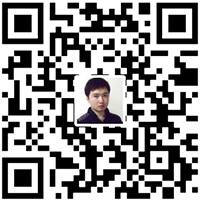# 实现文本相似度算法（余弦定理）

``````public class CosineSimilarAlgorithm {
public static double getSimilarity(String doc1, String doc2) {
if (doc1 != null && doc1.trim().length() > 0 && doc2 != null
&& doc2.trim().length() > 0) {

Map<Integer, int[]> AlgorithmMap = new HashMap<Integer, int[]>();

//将两个字符串中的中文字符以及出现的总数封装到，AlgorithmMap中
for (int i = 0; i < doc1.length(); i++) {
char d1 = doc1.charAt(i);
if(isHanZi(d1)){
int charIndex = getGB2312Id(d1);
if(charIndex != -1){
int[] fq = AlgorithmMap.get(charIndex);
if(fq != null && fq.length == 2){
fq++;
}else {
fq = new int;
fq = 1;
fq = 0;
AlgorithmMap.put(charIndex, fq);
}
}
}
}

for (int i = 0; i < doc2.length(); i++) {
char d2 = doc2.charAt(i);
if(isHanZi(d2)){
int charIndex = getGB2312Id(d2);
if(charIndex != -1){
int[] fq = AlgorithmMap.get(charIndex);
if(fq != null && fq.length == 2){
fq++;
}else {
fq = new int;
fq = 0;
fq = 1;
AlgorithmMap.put(charIndex, fq);
}
}
}
}

Iterator<Integer> iterator = AlgorithmMap.keySet().iterator();
double sqdoc1 = 0;
double sqdoc2 = 0;
double denominator = 0;
while(iterator.hasNext()){
int[] c = AlgorithmMap.get(iterator.next());
denominator += c*c;
sqdoc1 += c*c;
sqdoc2 += c*c;
}

return denominator / Math.sqrt(sqdoc1*sqdoc2);
} else {
throw new NullPointerException(
" the Document is null or have not cahrs!!");
}
}

public static boolean isHanZi(char ch) {
// 判断是否汉字
return (ch >= 0x4E00 && ch <= 0x9FA5);

}

/**
* 根据输入的Unicode字符，获取它的GB2312编码或者ascii编码，
*
* @param ch
*            输入的GB2312中文字符或者ASCII字符(128个)
* @return ch在GB2312中的位置，-1表示该字符不认识
*/
public static short getGB2312Id(char ch) {
try {
byte[] buffer = Character.toString(ch).getBytes("GB2312");
if (buffer.length != 2) {
// 正常情况下buffer应该是两个字节，否则说明ch不属于GB2312编码，故返回'?'，此时说明不认识该字符
return -1;
}
int b0 = (int) (buffer & 0x0FF) - 161; // 编码从A1开始，因此减去0xA1=161
int b1 = (int) (buffer & 0x0FF) - 161; // 第一个字符和最后一个字符没有汉字，因此每个区只收16*6-2=94个汉字
return (short) (b0 * 94 + b1);
} catch (UnsupportedEncodingException e) {
e.printStackTrace();
}
return -1;
}
}``````

1. 只将汉字作为向量，其他的如标点，数字等符号不处理；

2. 在HashMap中存放汉字和其在文本中对于的个数时，先将单个汉字通过GB2312编码转换成数字，再存放。支付宝微信